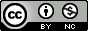## The many flavors of apply

September 13, 2010One of the more powerful capabilities of R is the “apply” family. These are functions whose purpose is to take an R function and some R object that represents “a set of things” and apply the function to each element in the set. You can often achieve the same results with a for loop, stepping through the elements of the set one by one, but the equivalent *apply commands are (1) more compact, making code easier to read [at least if you understand them!], (2) slightly more convenient — various bookkeeping such as figuring out the number of elements in the set and setting aside storage for the results gets done automatically, (3) more “idiomatic” in R (in case that matters to you), and (4) [sometimes] more efficient [although it is no longer always the case, as it was in early versions of S-PLUS, that for loops are much less efficient than the apply commands].

This general approach to programming (define a function, then apply it to a set of objects) is called (not too surprisingly) functional programming (http://en.wikipedia.org/wiki/Functional_programming). This style of programming started out in LISP, and is also very common in Mathematica (where it is represented by the Map function).

*applying is easiest when an existing function does what you want, but you can also define functions on the fly. For example, R doesn’t have a square() function. You could define it:

> square <- function(x) {
x^2
}
> sapply(1:5,square)
  1  4  9 16 25

but for this kind of short function you can just say

> sapply(1:5,function(x) {x^2})
  1  4  9 16 25

(Mathematica has an even slicker way to do this.)

You can also omit the curly brackets when your function consists of a single statement. If it has more than one you can use semicolons to keep all the statements on the same line, for compactness; e.g.

> sapply(1:5,function(x) {y <- x; y^2})
  1  4  9 16 25

(although in this case the extra statement is obviously pointless).

You’d also be surprised sometimes what can be used as a function:

> sapply(1:5,"^",2)
  1  4  9 16 25

This example also represents a powerful and sometimes overlooked feature of *apply: extra arguments get passed through to the function you are applying. This is particularly handy when you want to apply the function to a vector but use the vector as something other than the first argument to the function. For example, suppose we wanted to run a linear regression on a series of different data sets. Rather than

> datlist = list(dat1,dat2,dat3)
> lapply(datlist, function(d) lm(y~x,data=d))

we could just say

> datlist = list(dat1,dat2,dat3)
> lapply(datlist, lm, formula=y~x)

R will fill in the formula argument and then use the elements of datlist for the next unfilled argument, which in this case is data.

Note that applying can also be overdone: See section 4 of Patrick Burns’ “R Inferno” (http://www.burns-stat.com/pages/Tutor/R_inferno.pdf) (which is a pleasure to read in general).

Reproduced and slightly extended from that reference:

 function input output comment apply matrix or array vector or array or list lapply list or vector list sapply list or vector vector or matrix or list simplify tapply data, categories array or list ragged mapply lists and/or vectors vector or matrix or list multiple rapply list vector or list recursive eapply environment list dendrapply dendogram dendogram zoo::rollapply data similar to input emdbook::apply2d two vectors matrix multicore::mclapply same as lapply same as lapply parallelize across cores (OK on Unix, experimental for Windows (pre-Vista only): see http://rforge.net/multicore)

kernapply has the same pattern, but I don’t think it is really in the *apply family.

Also: simFrame::simApply, functions in Rmpi (mpi.parapply, mpi.iapply, mpi.apply), gridR::apply, RMySQL::dbApply, RPostgreSQL::dbApply, PerformanceAnalytics::apply.rolling, ff::ffapply, xts::{period.apply,apply.monthly}, etc. etc. etc.. (these are the results of sos::findFn("apply")). Also nlme::gapply.

### 1 apply

Apply fun to the “margins” of a matrix or array. “Margin” here means row, column, or other “slices” of a higher-dimensional array. The MARGIN argument is 1 for rows, 2 for columns, and n for another dimension of a higher-dimensional array. You can give more than one margin:

> m = matrix(1:4,byrow=TRUE,ncol=2)
> apply(m,c(1,2),function(x) x^2)

[,1] [,2]
[1,]    1    4
[2,]    9   16

Of course, in this case we don’t do any better than just saying m^2. But we could apply over more than one, but not all, dimensions of an array with > 2 dimensions.

colSums, rowSums, colMeans, rowMeans are special cases that are considerably faster than the equivalent apply commands. (I think there’s an equivalent for the median somewhere in a Bioconductor package.)

### 2 lapply

Apply a function to a list.

### 3 sapply

Apply a function to a list, or a vector (this is handy so you don’t have to say lapply(as.list(x)), and simplify the results if possible.

### 4 mapply

Apply a function of multiple arguments to multiple lists. I sometimes use this as a shortcut where I should probably just give up and use a for loop.

> mapply(function(dat,i) {
plot(dat\$x,dat\$y,col=i)
text(1,2,names(dat)[i])
},
datlist,1:length(datlist))

it would be great to have a way within an *apply function to access the current value of the index (or name of the current element) but I don’t know of one …

Additional arguments have to be specified explicitly with MoreArgs. Depending on what you’re doing you may want SIMPLIFY to be TRUE or FALSE

### Related functions

 function purpose do.call apply a function to a list of arguments replicate repeat an expression many times outer apply a function to all combinations of two vectors (function must be vectorized — otherwise see emdbook::apply2d Map equivalent to mapply: see ?funprog Reduce apply a function to successively combine elements cumsum (and cummax, cummin, cumprod): cumulative functions plyr::ddply (and friends) split an object, apply a function to chunks, then recombine the chunks (split/tapply/rbind on steroids)

For the truly clever: why does this work?

> N <- 0; replicate(20,N <<- N-round(0.25*N)+10)

 10 18 24 28 31 33 35 36 37 38 38 38 38 38 38 38 38 38 38 38